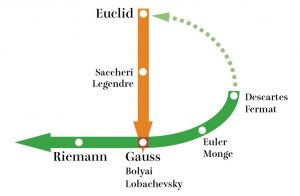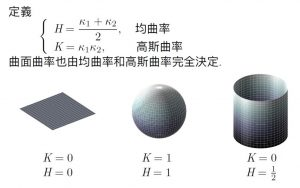非欧几何是一个时代的结束？还是开始？

If you ask a mathematician “why?” the mathematician will give you a proof.
But when you ask a historian “why?” the historian will tell you a story.

设准 vs. 公理

Postulate = Special Notion vs. Common Notion = Axiom

We will raise this conjecture (the purport of which will hereafter be called the “Principle of Relativity”) to the status of a postulate, and also introduce another postulate, which is only apparently irreconcilable with the former, namely, that light is always propagated in empty space with a definite velocity:c which is independent of the state of motion of the emitting body. These two postulates suffice for the attainment of a simple and consistent theory of the electrodynamics of moving bodies based on Maxwell’s theory for stationary bodies. The introduction of a “luminiferous aether” will prove to be superfluous inasmuch as the view here to be developed will not require an “absolutely stationary space” provided with special properties, nor assign a velocity-vector to a point of the empty space in which electromagnetic processes take place.

数学真理的相关议题

“Snow is white” is true if snow is white.

爱因斯坦的反思！

Morris Kline在《数学：确定性的失落》中写道：「倘若数学命题是对现实(reality)的描述，它们就不是确定的(certain)；倘若它们是确定的，那它们就不是描述现实。……然而另一方面，可以确定的是，不论就数学整体或是单就几何而言，它们的存在都是我们想要得知实际物体的性质。

非欧几何的历史

15世纪文艺复兴绘画开始发展的「透视」和「无穷远点」等射影几何概念，不被想成「非」欧氏几何，而是关联于欧氏几何发展的领域。

1. 绝对欧氏空间的扬弃，把实际空间还给物理学，把欧氏几何想成一种可能的几何（最简单的）。
2. 非欧几何必须相应处理原来欧氏几何的几何概念：长度、角度、面积、体积等。高斯曲率是内禀的几何量测量高斯曲率

Gauss-Bonnett定理之原版：三角形内角和

$\theta_1 + \theta_2+ \theta_3=\iint_{\triangle} K\mathrm dA.$

平面几何的准备

两圆正交

• 记 $$A$$ 是 $$\odot O_1$$ 与 $$\odot O_2$$ 的一个交点, 过 $$A$$ 分别作两圆的切线, 如果两切线垂直, 即 $$AO_1\perp AO_2$$, 则称这两圆为正交圆, 或称这两个圆正交.
• 一条直线如果经过一个圆的圆心, 称这直线与圆正交.

中文

1. 李忠, 周建莹, 双曲几何, 湖南教育出版社, 1991, 12
2. 项武义, 基础几何学, 人民教育出版社, 2004, 9
3. 项武义, 王申怀, 潘养廉, 古典几何学, 高等教育出版社, 2014, 5
4. 球面上的几何, 人民教育出版社, A 版, B 版
5. 李忠, 并不神秘的非欧几何, 高等教育出版社, 2010, 6
6. 王宗儒, 三角形的内角和等于 $$180^\circ$$ 吗?, 湖南人民出版社, 1981, 7

英文

1. Riccardo Benedetti, Carlo Petronio, Lectures on Hyperbolic Geometry, 2003, 7
2. Birger Iversen, Hyperbolic Geometry, Cambridge University Press, 1992, 12
3. J. W. Bruce, Linda Keen, Nikola Lakic, Hyperbolic Geometry from a Local Viewpoint. Cambridge University Press, 2007, 03